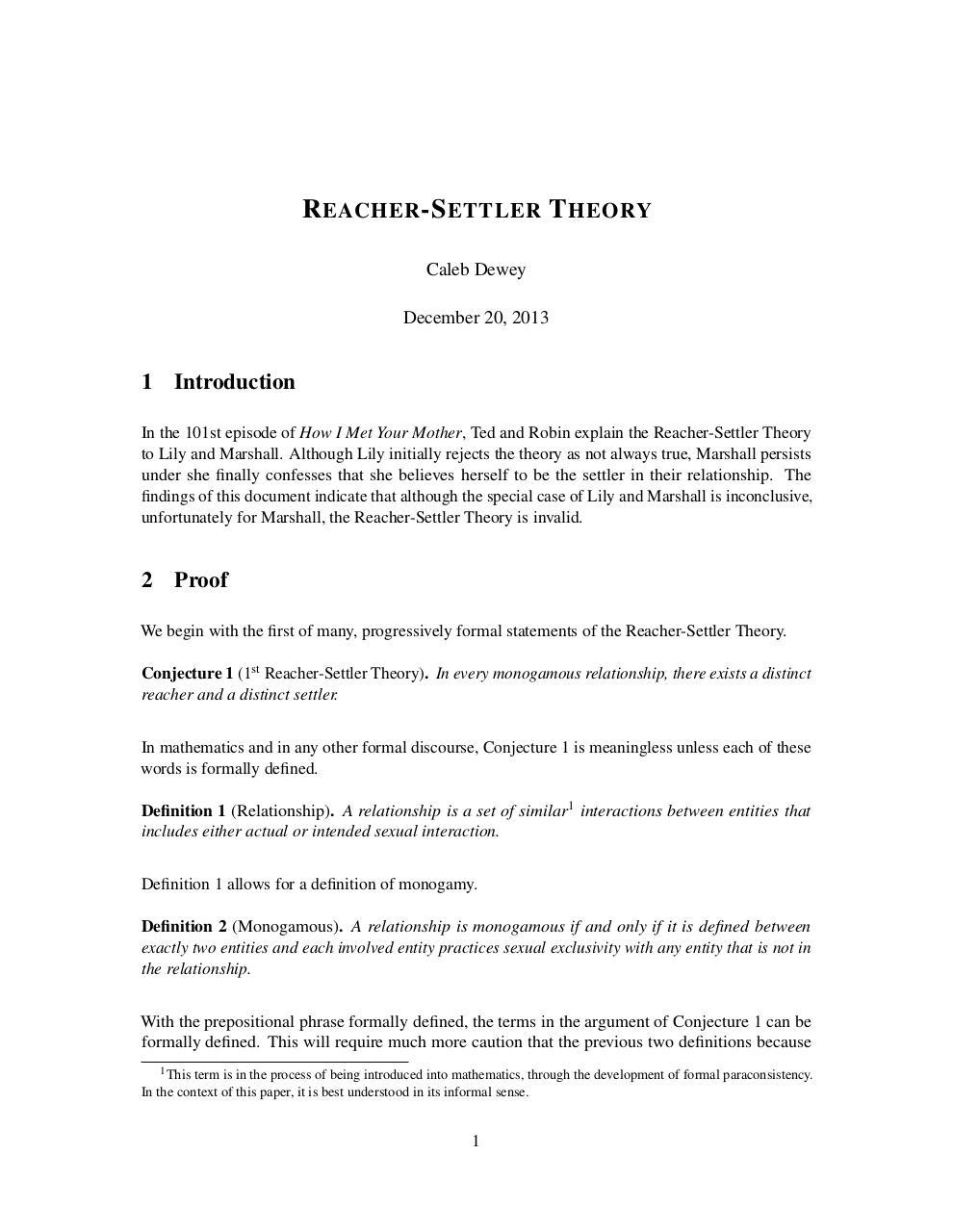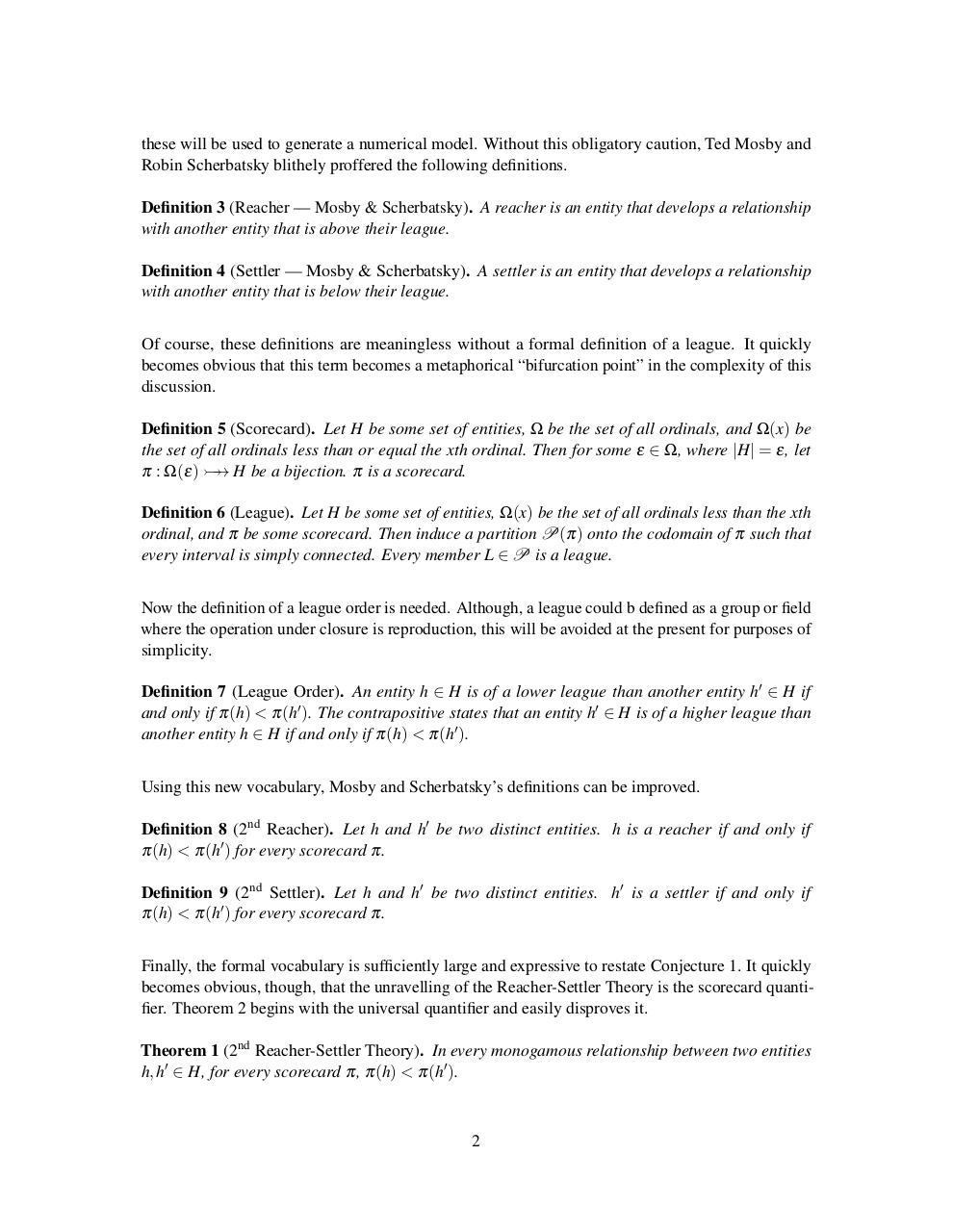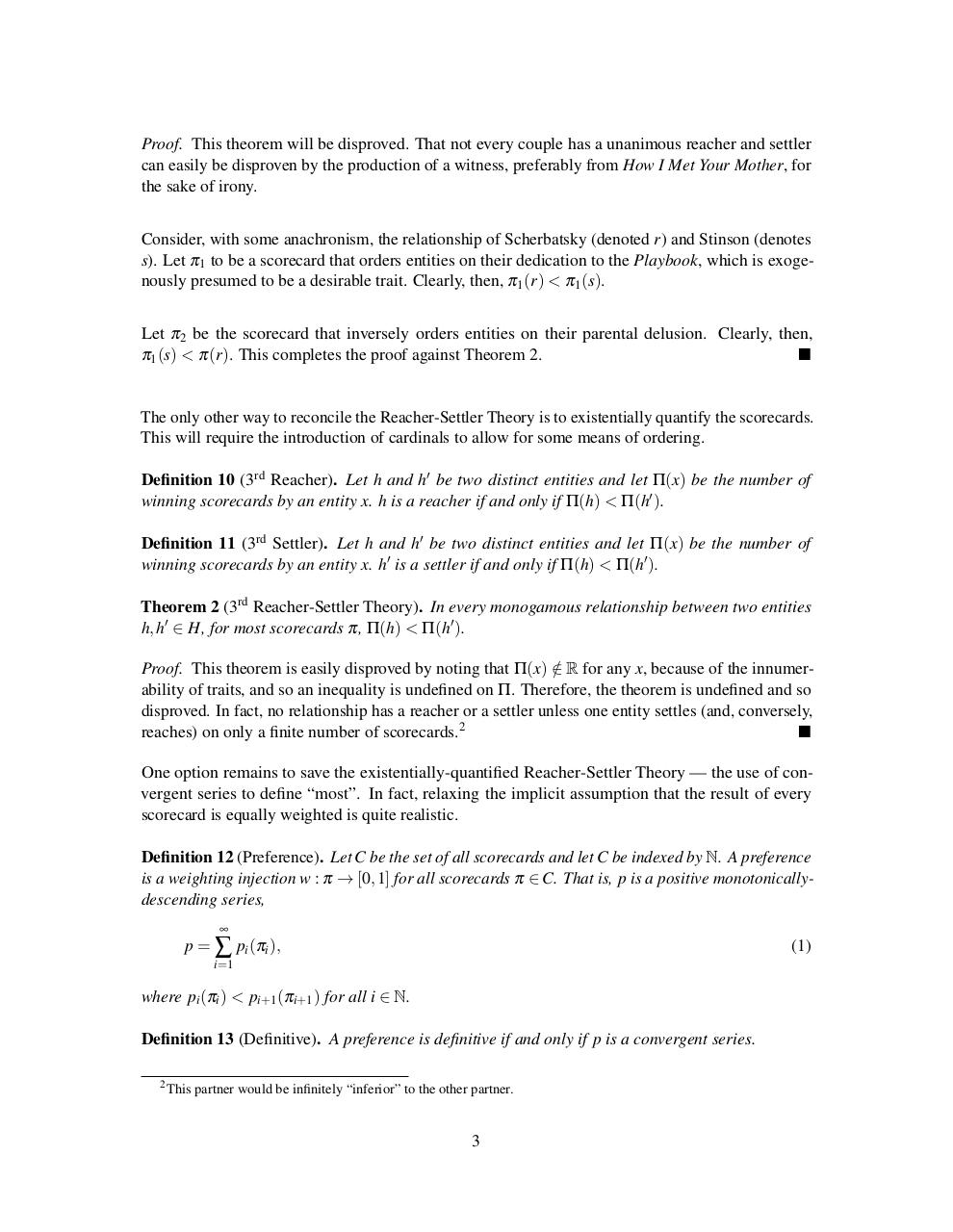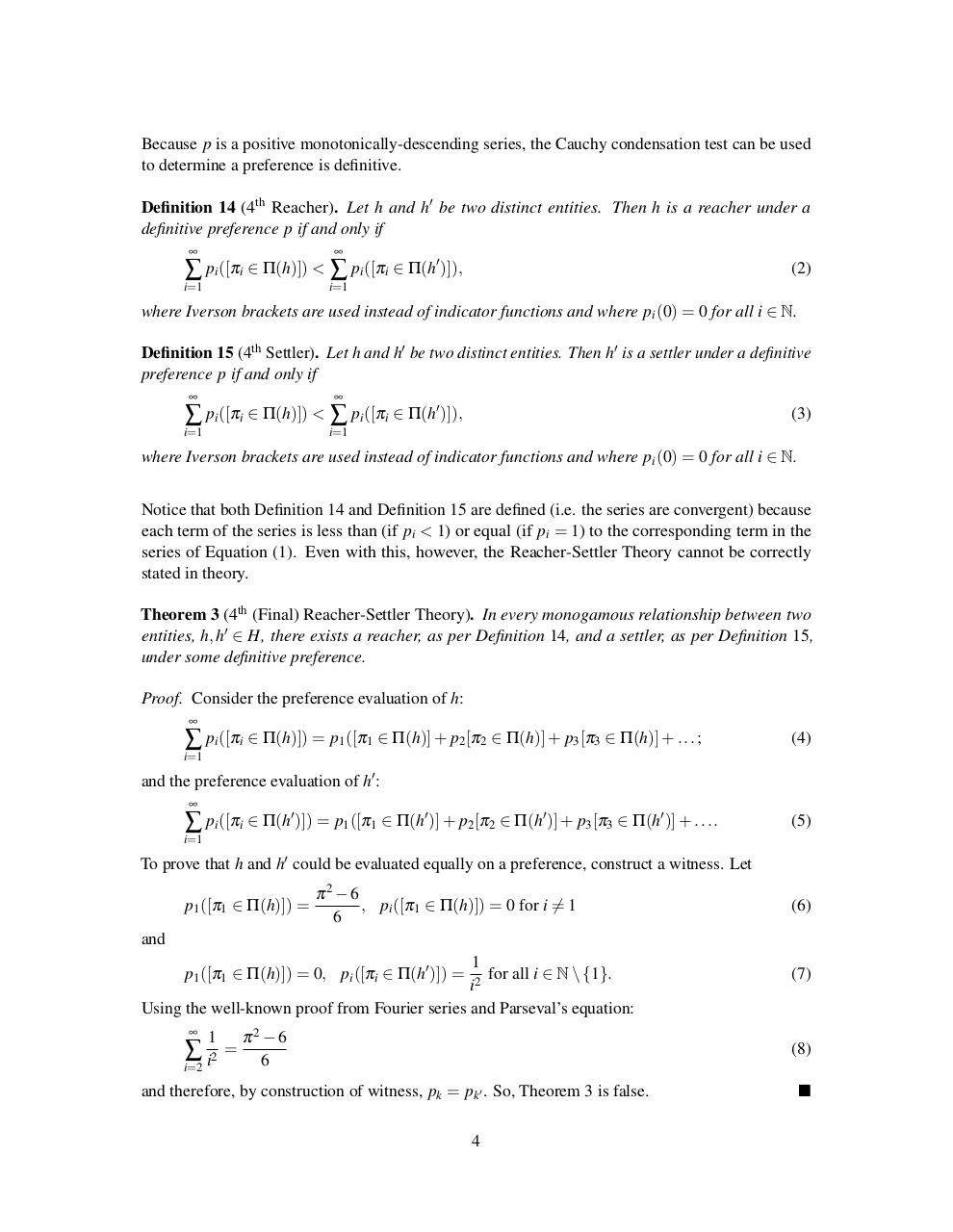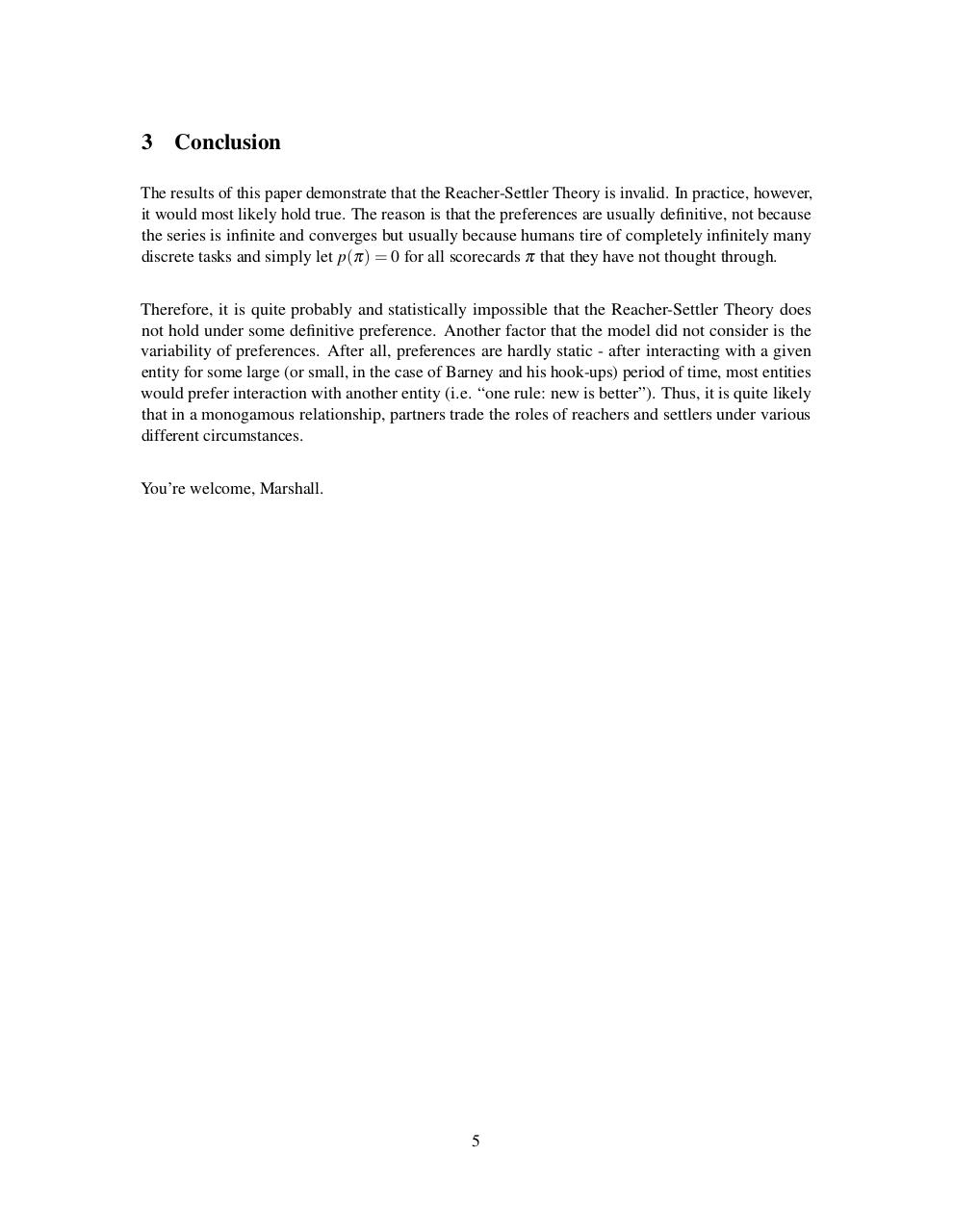# Reacher Settler Theory .pdf

### File information

Original filename: Reacher-Settler Theory.pdf

This PDF 1.5 document has been generated by TeX / pdfTeX-1.40.13, and has been sent on pdf-archive.com on 20/12/2013 at 20:44, from IP address 198.96.x.x. The current document download page has been viewed 1022 times.
File size: 98 KB (5 pages).
Privacy: public file

Reacher-Settler Theory.pdf (PDF, 98 KB)

### Document preview

R EACHER -S ETTLER T HEORY
Caleb Dewey
December 20, 2013

1

Introduction

In the 101st episode of How I Met Your Mother, Ted and Robin explain the Reacher-Settler Theory
to Lily and Marshall. Although Lily initially rejects the theory as not always true, Marshall persists
under she finally confesses that she believes herself to be the settler in their relationship. The
findings of this document indicate that although the special case of Lily and Marshall is inconclusive,
unfortunately for Marshall, the Reacher-Settler Theory is invalid.

2

Proof

We begin with the first of many, progressively formal statements of the Reacher-Settler Theory.
Conjecture 1 (1st Reacher-Settler Theory). In every monogamous relationship, there exists a distinct
reacher and a distinct settler.
In mathematics and in any other formal discourse, Conjecture 1 is meaningless unless each of these
words is formally defined.
Definition 1 (Relationship). A relationship is a set of similar1 interactions between entities that
includes either actual or intended sexual interaction.
Definition 1 allows for a definition of monogamy.
Definition 2 (Monogamous). A relationship is monogamous if and only if it is defined between
exactly two entities and each involved entity practices sexual exclusivity with any entity that is not in
the relationship.
With the prepositional phrase formally defined, the terms in the argument of Conjecture 1 can be
formally defined. This will require much more caution that the previous two definitions because
1 This

term is in the process of being introduced into mathematics, through the development of formal paraconsistency.
In the context of this paper, it is best understood in its informal sense.

1

these will be used to generate a numerical model. Without this obligatory caution, Ted Mosby and
Robin Scherbatsky blithely proffered the following definitions.
Definition 3 (Reacher — Mosby &amp; Scherbatsky). A reacher is an entity that develops a relationship
with another entity that is above their league.
Definition 4 (Settler — Mosby &amp; Scherbatsky). A settler is an entity that develops a relationship
with another entity that is below their league.
Of course, these definitions are meaningless without a formal definition of a league. It quickly
becomes obvious that this term becomes a metaphorical “bifurcation point” in the complexity of this
discussion.
Definition 5 (Scorecard). Let H be some set of entities, Ω be the set of all ordinals, and Ω(x) be
the set of all ordinals less than or equal the xth ordinal. Then for some ε ∈ Ω, where |H| = ε, let
π : Ω(ε) 
→ H be a bijection. π is a scorecard.
Definition 6 (League). Let H be some set of entities, Ω(x) be the set of all ordinals less than the xth
ordinal, and π be some scorecard. Then induce a partition P(π) onto the codomain of π such that
every interval is simply connected. Every member L ∈ P is a league.
Now the definition of a league order is needed. Although, a league could b defined as a group or field
where the operation under closure is reproduction, this will be avoided at the present for purposes of
simplicity.
Definition 7 (League Order). An entity h ∈ H is of a lower league than another entity h0 ∈ H if
and only if π(h) &lt; π(h0 ). The contrapositive states that an entity h0 ∈ H is of a higher league than
another entity h ∈ H if and only if π(h) &lt; π(h0 ).
Using this new vocabulary, Mosby and Scherbatsky’s definitions can be improved.
Definition 8 (2nd Reacher). Let h and h0 be two distinct entities. h is a reacher if and only if
π(h) &lt; π(h0 ) for every scorecard π.
Definition 9 (2nd Settler). Let h and h0 be two distinct entities. h0 is a settler if and only if
π(h) &lt; π(h0 ) for every scorecard π.
Finally, the formal vocabulary is sufficiently large and expressive to restate Conjecture 1. It quickly
becomes obvious, though, that the unravelling of the Reacher-Settler Theory is the scorecard quantifier. Theorem 2 begins with the universal quantifier and easily disproves it.
Theorem 1 (2nd Reacher-Settler Theory). In every monogamous relationship between two entities
h, h0 ∈ H, for every scorecard π, π(h) &lt; π(h0 ).

2

Proof. This theorem will be disproved. That not every couple has a unanimous reacher and settler
can easily be disproven by the production of a witness, preferably from How I Met Your Mother, for
the sake of irony.
Consider, with some anachronism, the relationship of Scherbatsky (denoted r) and Stinson (denotes
s). Let π1 to be a scorecard that orders entities on their dedication to the Playbook, which is exogenously presumed to be a desirable trait. Clearly, then, π1 (r) &lt; π1 (s).
Let π2 be the scorecard that inversely orders entities on their parental delusion. Clearly, then,
π1 (s) &lt; π(r). This completes the proof against Theorem 2.


The only other way to reconcile the Reacher-Settler Theory is to existentially quantify the scorecards.
This will require the introduction of cardinals to allow for some means of ordering.
Definition 10 (3rd Reacher). Let h and h0 be two distinct entities and let Π(x) be the number of
winning scorecards by an entity x. h is a reacher if and only if Π(h) &lt; Π(h0 ).
Definition 11 (3rd Settler). Let h and h0 be two distinct entities and let Π(x) be the number of
winning scorecards by an entity x. h0 is a settler if and only if Π(h) &lt; Π(h0 ).
Theorem 2 (3rd Reacher-Settler Theory). In every monogamous relationship between two entities
h, h0 ∈ H, for most scorecards π, Π(h) &lt; Π(h0 ).
Proof. This theorem is easily disproved by noting that Π(x) ∈
/ R for any x, because of the innumerability of traits, and so an inequality is undefined on Π. Therefore, the theorem is undefined and so
disproved. In fact, no relationship has a reacher or a settler unless one entity settles (and, conversely,
reaches) on only a finite number of scorecards.2

One option remains to save the existentially-quantified Reacher-Settler Theory — the use of convergent series to define “most”. In fact, relaxing the implicit assumption that the result of every
scorecard is equally weighted is quite realistic.
Definition 12 (Preference). Let C be the set of all scorecards and let C be indexed by N. A preference
is a weighting injection w : π → [0, 1] for all scorecards π ∈ C. That is, p is a positive monotonicallydescending series,

p = ∑ pi (πi ),

(1)

i=1

where pi (πi ) &lt; pi+1 (πi+1 ) for all i ∈ N.
Definition 13 (Definitive). A preference is definitive if and only if p is a convergent series.
2 This

partner would be infinitely “inferior” to the other partner.

3

Because p is a positive monotonically-descending series, the Cauchy condensation test can be used
to determine a preference is definitive.
Definition 14 (4th Reacher). Let h and h0 be two distinct entities. Then h is a reacher under a
definitive preference p if and only if

i=1

i=1

∑ pi ([πi ∈ Π(h)]) &lt; ∑ pi ([πi ∈ Π(h0 )]),

(2)

where Iverson brackets are used instead of indicator functions and where pi (0) = 0 for all i ∈ N.
Definition 15 (4th Settler). Let h and h0 be two distinct entities. Then h0 is a settler under a definitive
preference p if and only if

i=1

i=1

∑ pi ([πi ∈ Π(h)]) &lt; ∑ pi ([πi ∈ Π(h0 )]),

(3)

where Iverson brackets are used instead of indicator functions and where pi (0) = 0 for all i ∈ N.
Notice that both Definition 14 and Definition 15 are defined (i.e. the series are convergent) because
each term of the series is less than (if pi &lt; 1) or equal (if pi = 1) to the corresponding term in the
series of Equation (1). Even with this, however, the Reacher-Settler Theory cannot be correctly
stated in theory.
Theorem 3 (4th (Final) Reacher-Settler Theory). In every monogamous relationship between two
entities, h, h0 ∈ H, there exists a reacher, as per Definition 14, and a settler, as per Definition 15,
under some definitive preference.
Proof. Consider the preference evaluation of h:

∑ pi ([πi ∈ Π(h)]) = p1 ([π1 ∈ Π(h)] + p2 [π2 ∈ Π(h)] + p3 [π3 ∈ Π(h)] + . . . ;

(4)

i=1

and the preference evaluation of h0 :

∑ pi ([πi ∈ Π(h0 )]) = p1 ([π1 ∈ Π(h0 )] + p2 [π2 ∈ Π(h0 )] + p3 [π3 ∈ Π(h0 )] + . . . .

(5)

i=1

To prove that h and h0 could be evaluated equally on a preference, construct a witness. Let
p1 ([π1 ∈ Π(h)]) =

π2 − 6
, pi ([π1 ∈ Π(h)]) = 0 for i 6= 1
6

(6)

and
1
for all i ∈ N \ {1}.
i2
Using the well-known proof from Fourier series and Parseval’s equation:
p1 ([π1 ∈ Π(h)]) = 0, pi ([πi ∈ Π(h0 )]) =

1

∑ i2 =

i=2

π2 − 6
6

(7)

(8)

and therefore, by construction of witness, pk = pk0 . So, Theorem 3 is false.
4



3

Conclusion

The results of this paper demonstrate that the Reacher-Settler Theory is invalid. In practice, however,
it would most likely hold true. The reason is that the preferences are usually definitive, not because
the series is infinite and converges but usually because humans tire of completely infinitely many
discrete tasks and simply let p(π) = 0 for all scorecards π that they have not thought through.
Therefore, it is quite probably and statistically impossible that the Reacher-Settler Theory does
not hold under some definitive preference. Another factor that the model did not consider is the
variability of preferences. After all, preferences are hardly static - after interacting with a given
entity for some large (or small, in the case of Barney and his hook-ups) period of time, most entities
would prefer interaction with another entity (i.e. “one rule: new is better”). Thus, it is quite likely
that in a monogamous relationship, partners trade the roles of reachers and settlers under various
different circumstances.
You’re welcome, Marshall.

5